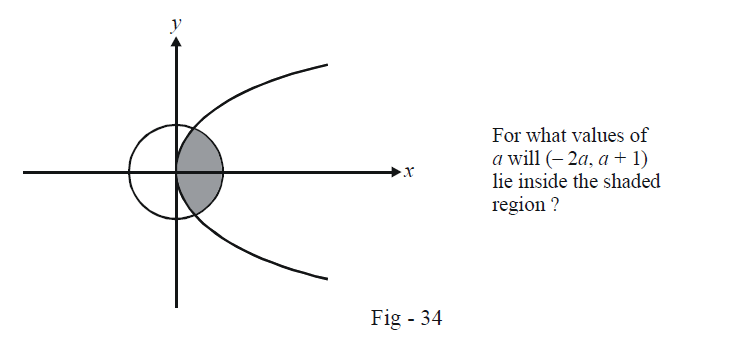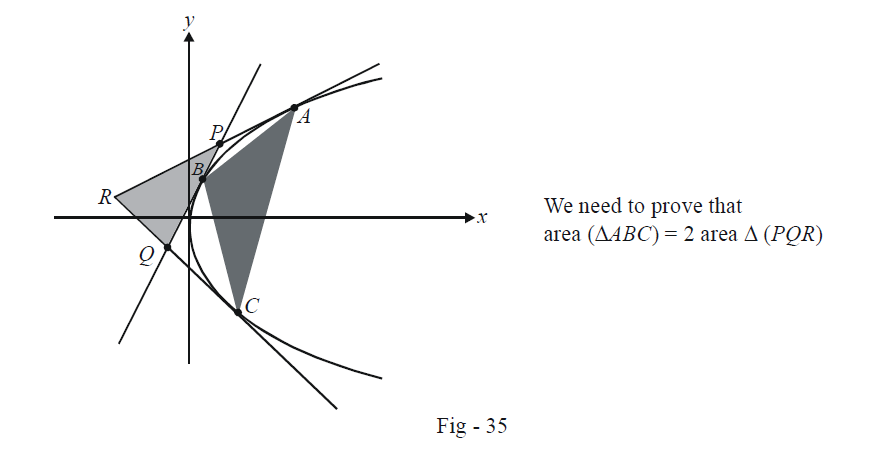# Parabolas Set 1

Go back to  'SOLVED EXAMPLES'

Example - 1

Find the values that a can take so that the point $$P\,( - 2a,\,\,a + 1)$$ lies inside the smaller region bounded by the circle $${x^2} + {y^2} =4$$ and the parabola $${y^2} = 4x$$ as shown:Solution: Note that the shaded region represents the interior of both the circle and the parabola. Thus,

\begin{align}&\qquad \quad \underbrace {{{( - 2a)}^2} + {{(a + 1)}^2} - 4 < 0}_{{\text{so that }}P{\text{ lies in the}} \atop {\text{interior of the circle}}} \quad{\rm{and}} \quad\underbrace {{{(a + 1)}^2} - 4( - 2a) < 0}_{{\text{so that }}P{\text{ lies in the}} \atop {\text{interior of the parabola}} }\\\\&\Rightarrow \qquad 5{a^2} + 2a - 3 < 0 \quad {\rm{and}}\quad {a^2} + 10a + 1 < 0\\\\&\Rightarrow \qquad- 1 < a < \frac{3}{5} \quad {\rm{and}} \quad - \sqrt 5 - 2\sqrt 6 < a < - 5 + 2\sqrt 6\end{align}

The intersection of the two constraints gives the possible values of a as

$a \in ( - 1,\, - 5 + 2\sqrt 6 )$

Example – 2

Prove that the area of the triangle formed by three points on a parabola is twice thearea of the triangle formed by the tangents at these points.

Solution:Let the parabola be $${y^2} = 4ax$$ .

Assume the points A, B and C to be $${t_1},{\rm{ }}{t_2}\;and\;{\rm{ }}{t_3}$$ respectively. Thus, P, Q and R are respectively $$(a{t_1}{t_2},\,a({t_1} +{t_2})),\,\,(a{t_2}{t_3},\,a({t_2} + {t_3})){\rm{ }}\,{\rm{and}}\,{\rm{ }}(a{t_3}{t_1},\,a({t_3}+ {t_1})).$$ Now we simply use the determinant formula to find the area of the two triangles

\begin{align}{\rm{area}}\;(\Delta ABC) &=\frac{1}{2}\left| {\begin{array}{*{20}{c}}{at_1^2}&{at_2^2}&{at_3^2}\\{2a{t_1}}&{2a{t_2}}&{2a{t_3}}\\1&1&1\end{array}}\right|\\\\&= {a^2}\left|{\begin{array}{*{20}{c}}{t_1^2}&{t_2^2 -t_1^2}&{t_3^2 - t_1^2}\\{{t_1}}&{{t_2} -{t_1}}&{{t_3} - {t_1}}\\1&0&0\end{array}}\right|{\rm{ }}\qquad\left\{ \begin{array}{l}{\rm{Using \;}}{C_2}\to {C_2} - {C_1};\\\,\,\,\,\,\,\,\,\,\,\,\,\,\;\;{C_3}\to {C_3} - {C_1}\end{array}\right\}\\\\&= {a^2}({t_2} -{t_1})({t_3} - {t_1})\left| {\begin{array}{*{20}{c}}{t_1^2}&{{t_2} +{t_1}}&{{t_3} + {t_1}}\\{{t_1}}&1&1\\1&0&0\end{array}}\right|\\\\&= - {a^2}({t_1} -{t_2})({t_2} - {t_3})({t_3} - {t_1})\end{align}

Similarly,

\begin{align} {\rm{area}} \;(\Delta PQR)= \frac{1}{2}\left| {\begin{array}{*{20}{c}} {a{t_1}{t_2}}&{a{t_2}{t_3}}&{a{t_3}{t_1}}\\ {a({t_1} + {t_2})}&{a({t_2} + {t_3})}&{a({t_3} + {t_1})}\\ 1&1&1\\\end{array}} \right|\\\\\begin{array}{l}&\;\;=\frac{{{a^2}}}{2}\left| {\begin{array}{*{20}{c}}{{t_1}{t_2}}&{{t_2}({t_3}- {t_1})}&{{t_1}({t_3} - {t_2})}\\{({t_1} +{t_2})}&{{t_3} - {t_1}}&{{t_3} - {t_2}}\\1&0&0\end{array}}\right|\\\\&\;\;=\frac{{{a^2}}}{2}({t_3} - {t_2})({t_3} - {t_1})\left| {\begin{array}{*{20}{c}}{{t_1}{t_2}}&{{t_2}}&{{t_1}}\\{({t_1} +{t_2})}&1&1\\1&0&0\end{array}}\right|\\\\&\;\;=\frac{{{a^2}}}{2}({t_2} - {t_1})({t_2} - {t_3})({t_3} - {t_1})\end{array} \end{align}

Clearly, $$\left| {{\rm{area }}(\Delta ABC)}\right| = 2\left| {{\rm{area }}(\Delta PQR)} \right|$$

This example should show you that the solution would have been much lengthier had wenot remembered the formula for the co-ordinates of the intersection point of two tangents. This is one subject where some things are to be learnt!

Learn from the best math teachers and top your exams

• Live one on one classroom and doubt clearing
• Practice worksheets in and after class for conceptual clarity
• Personalized curriculum to keep up with school